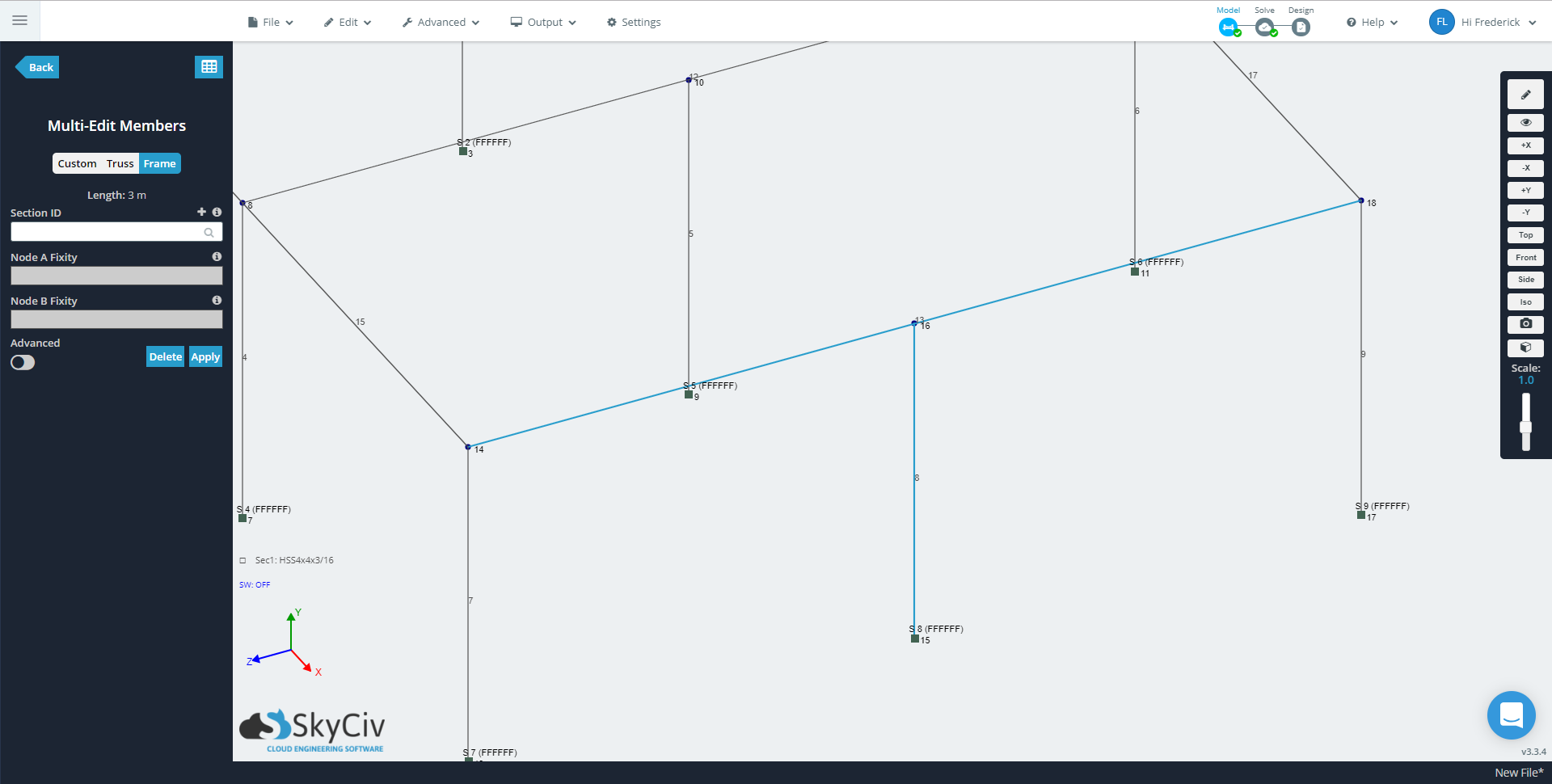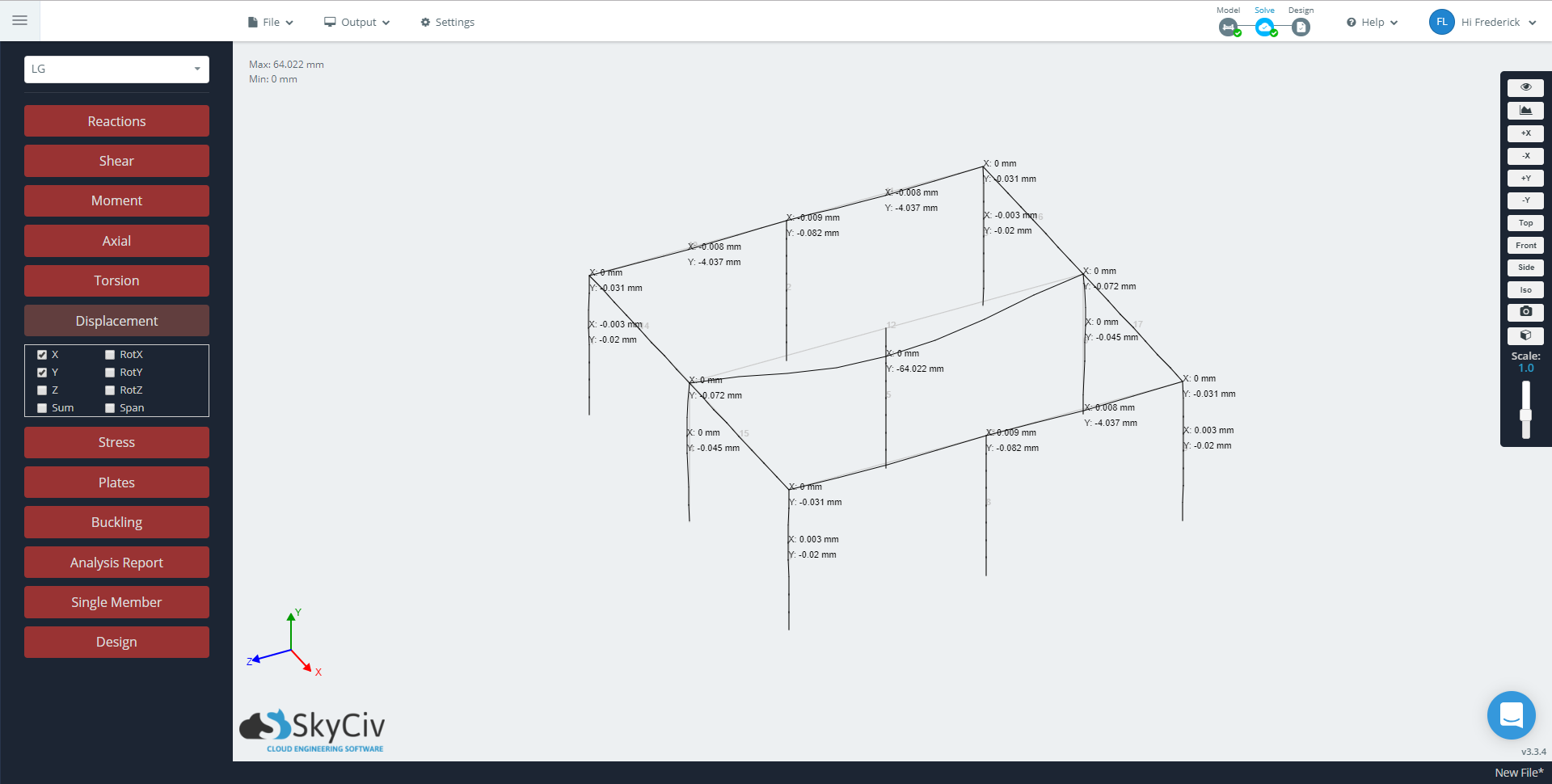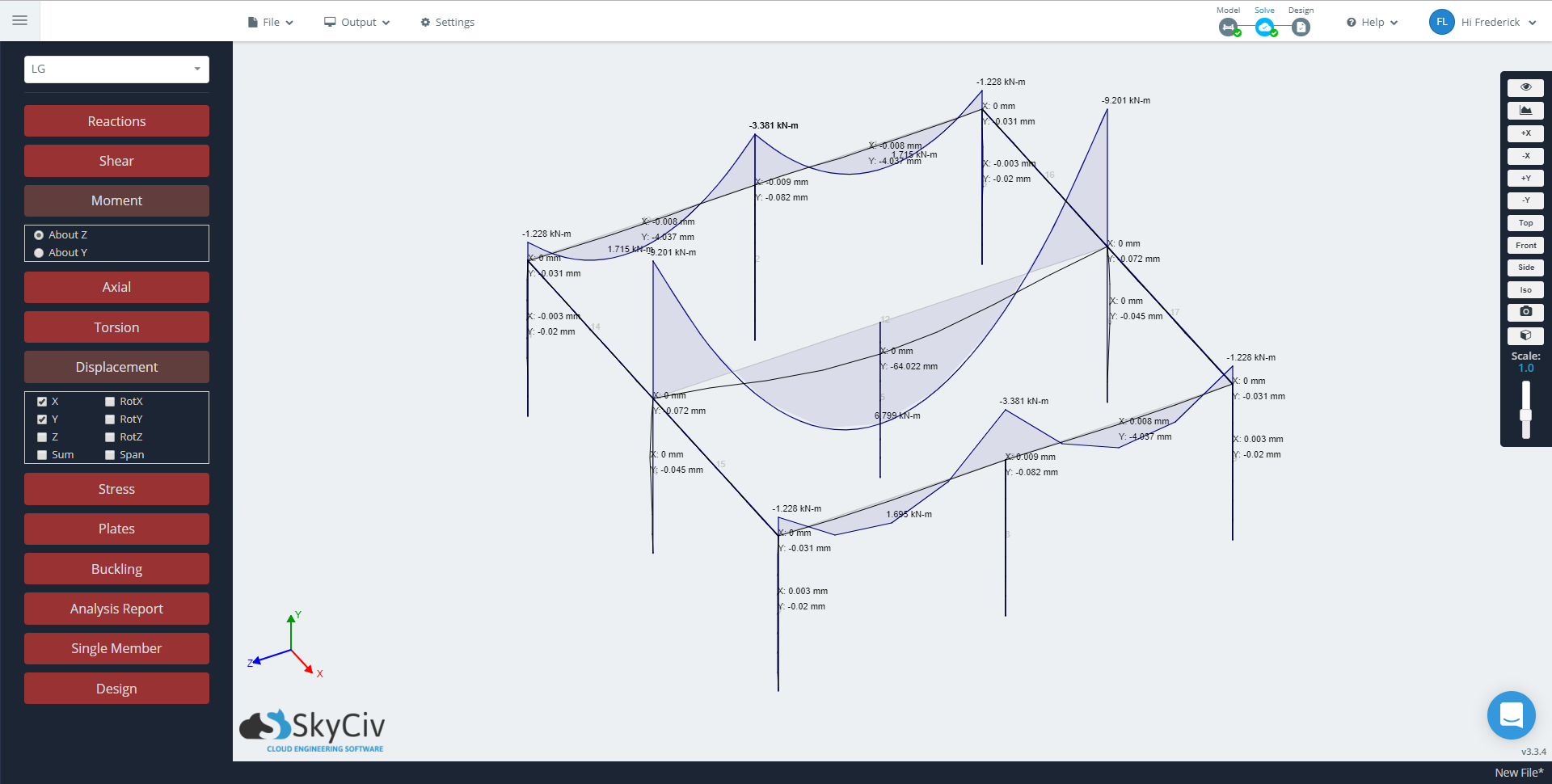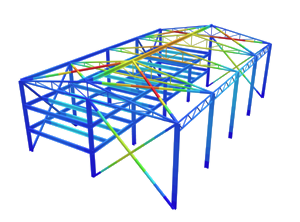SkyCiv Documentation

Your guide to SkyCiv software - tutorials, how-to guides and technical articles

1. Home
2. SkyCiv Structural 3D
3. Members
4. Normal vs Continuous Members

# Normal vs Continuous Members

## Continuous Members vs. Normal Members – when to use each

Summary: ‘Continuous’ Members are exactly like ‘Normal’ Members, except they automatically connect to any nodes that intersect the member.

### Introduction

This article will explore an in-depth look at how members (or elements) connect to each other via nodes and how this is handled in the SkyCiv Solver. Nodes mark a point of connectivity between members, they are the separation of the stiffness matrices.

Members can be a number of different types. In fact, Structural 3D actually has a current total of 6 member types. At the moment, we will look into the two types of members as these are fundamental member types for modeling in general. There are two main member types in Structural 3D: normal and continuous members. This discussion will be more in-depth with how they are both handled by the SkyCiv solver, as well as the advantages of using one from the other.

### Normal Members

Normal members are elements that are treated ‘normally’ in the sense that the members are analyzed in the traditional sense.

Traditionally, finite element analysis (FEA) solvers work by using the direct stiffness method. Based on the applied loads and stiffness of the elements in the structure, the displacement of the structure can be solved as shown in the following equation:

$$Q=KD$$

Where:

$$Q=$$ vector of stiffness

$$K=$$ global stiffness matrix (assembled from each member’s stiffness matrix)

$$D=$$ vector of unknown nodal displacements which are unknown and to be solved

SkyCiv Structural 3D applies these formulas to the model and obtains the results from every node and member.

### Continuous

Continuous members in Structural 3D are a type of member that allows for connectivity between two nodes to a node(s) that has no nodal connection to the member. Node connections occur when a node is assigned to connect two or more members.In the case shown below, the nodes are clearly not connected as the two members do not share a common node. Realistically they should be connected because the node of the second member (column) is along the path of the first member (beam).

The member deflecting the largest in the image below is selected as a ‘Normal’ member, therefore it does not recognize the column along its length. Changing the member to ‘Continuous’ will “connect” the long member to the column, which is the case for the other two members showing limited deflection.#### Normal Members

Models built with normal members are more likely to be compatible with third-party structural analysis software. As other programs may not support continuous members in the way that Structural 3D implements it, the results provided may not be correct.

It is important to check that there are no continuous members in the model before exporting it.

In terms of analysis, as the member is broken into simple members, the number of evaluation points in the model is more as opposed to continuous members which carry the same number of evaluation points across two or more spans, making the results less detailed. One can increase the number of evaluation points in the General Solver Settings, but as this controls the evaluation points for all members, the analysis could be longer. This can be a disadvantage when working with large and complex structures.#### Continuous Members

Continuous members, on the other hand, are very useful when it comes to increasing the efficiency in modeling. Continuous members reduce the number of members required to make a similar model with normal members. This directly impacts the amount of loads that will be present in your project. e.g., Loading a continuous member with a distributed load vs loading each normal member with a distributed load.

With respect to analysis, reducing the amount of members reduces the clutter and makes it easier to interpret and present the results. Having fewer members reduces the amount of subsequent model elements in your project, making it easier to see member behavior. For example, with continuous members, you can check the behavior over a larger span, rather than a combination of normal members over the same span.

When it comes to design, continuous members are great for reinforced concrete beams as they are intended to behave monolithically. As a span does not usually change its cross-section in-between, it is also useful in identifying the required steel section for a particular span. For example, continuous members are almost always recommended when designing girders or members that frame into them, i.e., floor or roof framing.